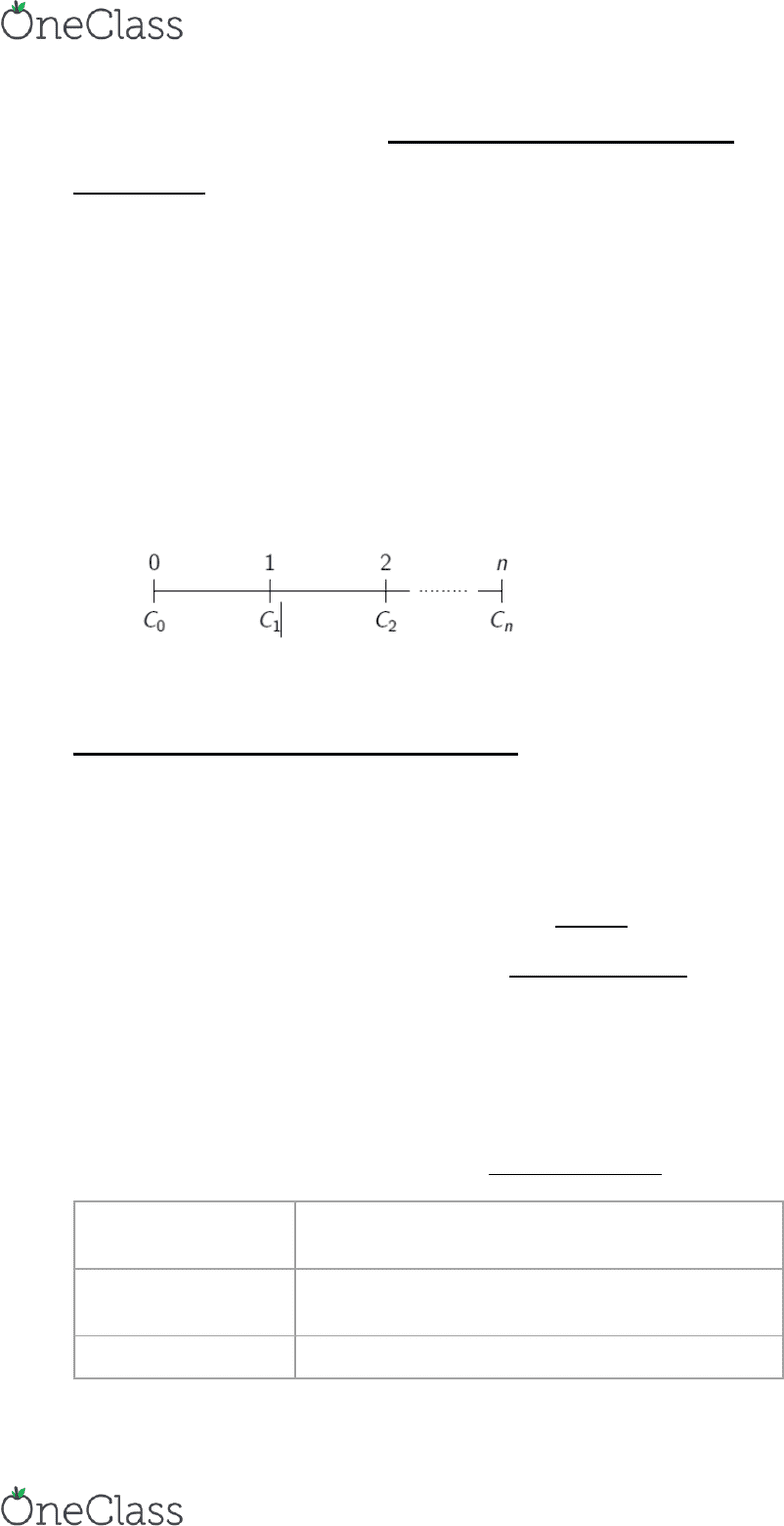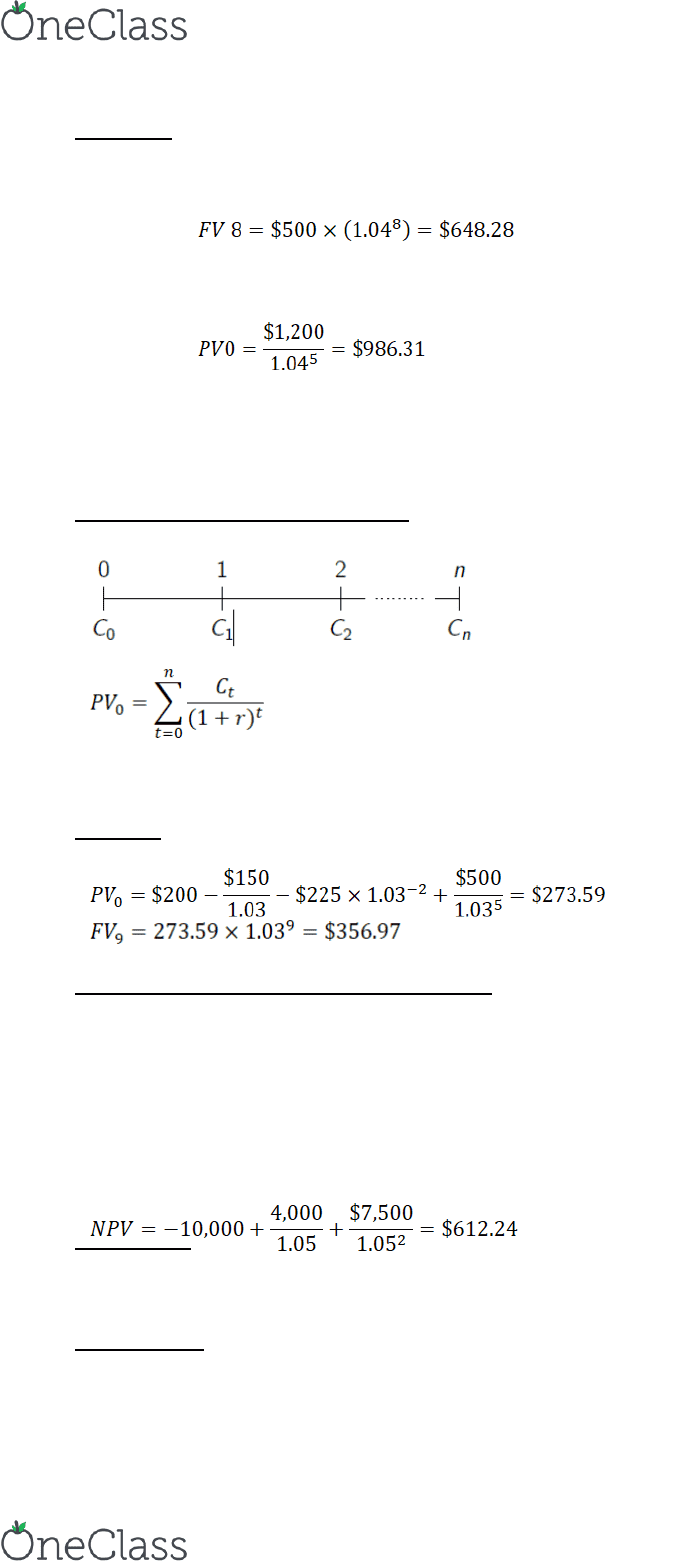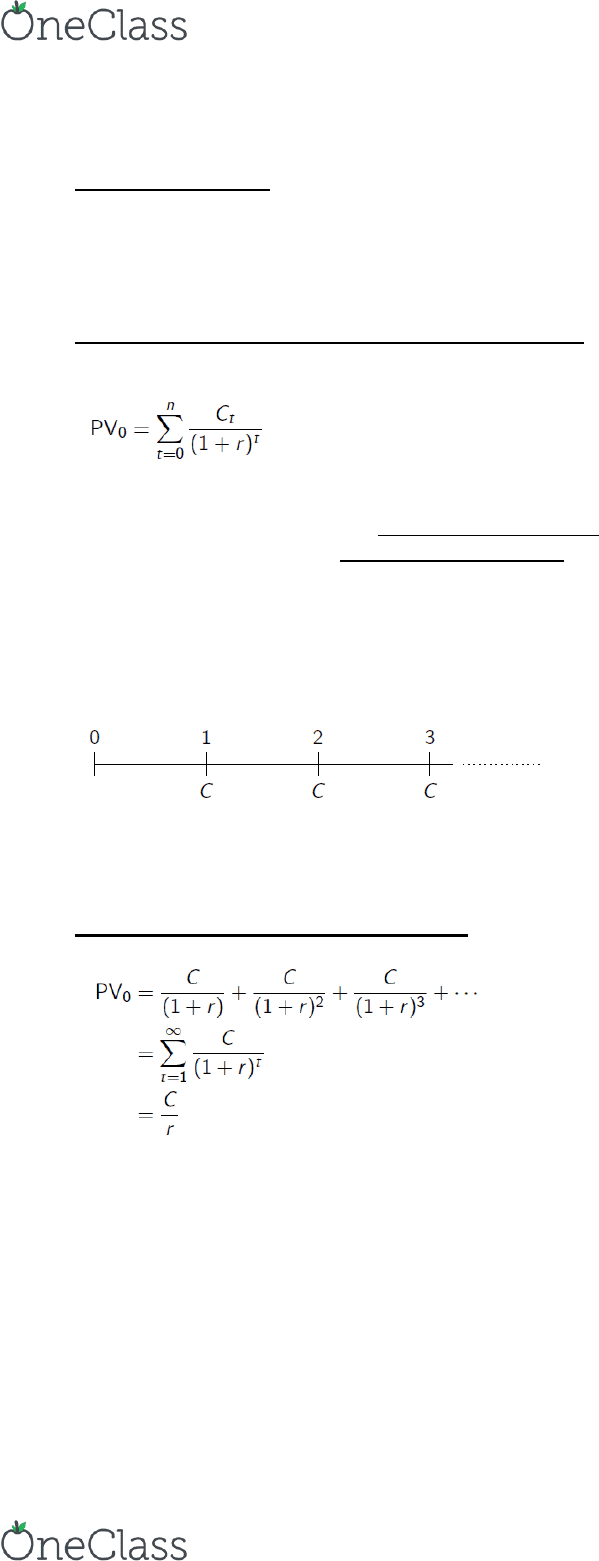# AFM 273 Chapter 4: C4: Time-Value-of-Money

83 views8 pages1
Ch. 4 Time Value of Money
The Timeline
A cash flow involves either receiving or paying money
o if money is received (a cash inflow), the sign of the cash flow is positive
o if money is paid (a cash outflow), the sign is negative
A stream of cash flows is a group of several cash flows occurring at different points in time
In general, the length of time between cash flow dates is called a “period
o A period could be different in terms of context
Depending on the particular context, a period could be a day, or a month, or a year, etc.
The times associated with a cash flow stream are date 0 (today), date 1 (one period from today),
date 2 (two periods from today), etc.
o The cash flows for these dates respectively are C0, C1, C2, etc.
A timeline can be used to represent a stream of cash flows
Each point on the timeline describes the beginning one period and the end of one period
o e.g. Date 1 is the end of Year 1 and beginning of Year 2
In general terms, with n periods:
A specific example: a friend agrees to lend you \$5,000 today and in return you agree to pay your
friend \$1,700 at the end of each of the next three months
3 Fundamental Rules of TVOM Calculations
Rule 1: Only cash flow values at the same point in time can be compared or combined
A dollar today and a dollar in 1 year are not equivalent
Cash flows must be converted into the same units or moved to the same point in time
Rule 2: To move a cash flow forward in time, compound it
Compounding: moving a value or cash flow forward in time
Let r denote the relevant interest rate per period
To compound a cash flow for n periods, multiply it by (1+r)n
Time value of money: the equivalent value of two cash flows at two different points in time
Simple interest: when an investment only earns interest on principal and no interest on accrued
interest
Compound interest: when an investment earns interest on principal and accrued interest
Geometric or exponential growth
Rule 3: To move a cash flow backward in time, discount it
To discount a cash flow for n periods, divide it by (1 + r)n
FV
(1+r)n
The value of a cash flow cash flow forward in
time
PV
The value today of a cash flow occurring in the
future, discount it to time 0
Discounted Value
The value of a cash flow moved backward in time
find more resources at oneclass.com
find more resources at oneclass.com
Unlock document

This preview shows pages 1-3 of the document.
Unlock all 8 pages and 3 million more documents.2
Examples
Suppose that r = 4% per year:
i. Consider an initial cash flow of C0 = \$500. What is the future value of this cash flow 8 years
from today?
ii. Consider instead a cash flow to be received 5 years from today of \$1,200. What is the present
value of this cash flow?
Text figure 4.1 breaks down the future value of an investment over time into the original amount
invested, interest received on this original amount, and interest on previously received interest
Valuing a Stream of Cash Flows
Suppose we want to determine the present value of a stream of cash flows C0, C1, C2 Cn:

Example
Suppose r = 3% and C0 = \$200, C1 = \$150, C2 = \$225, and C5 = \$500. Calculate PV0 and FV9.
Calculating the Net Present Value (NPV)
NPV = PV(benefits) PV(costs) this is equivalent to defining the NPV of an investment
Opportunity as the present value of the stream of cash flows of that opportunity
o Benefit cash inflow
o Cost cash outflow
Example: an investment that costs \$10,000 today will produce cash flows of \$4,000 one year from today
and \$7,500 two years from today. If the appropriate discount rate is 5%, should you make this
investment?
Spend now!
Borrow \$10,612.24 today for 2 years at 5% interest
o Invest \$10,000 and spend \$612.24
After 2 Years
You owe: \$10,612.24 x 1.052 = \$11,700
find more resources at oneclass.com
find more resources at oneclass.com
Unlock document

This preview shows pages 1-3 of the document.
Unlock all 8 pages and 3 million more documents.3
Investment generates \$4,000 x 1.05 + \$7,500 = \$11,700 after 2 years
--------------------------------------------------------------------------------
Spend After 2 years
Lend \$10,000 at 5% interest \$10,000 x 1.052 = \$11,025
Invest in project have \$11,700
Have extra \$675 \$612.24 x 1.052
Perpetuities, Annuities, and Other Special Cases
Recall that we can calculate the present value of a general cash flow stream by adding up the
present values of each of the cash flows, i.e.
In some cases a special pattern of cash flows allows us to use quicker formulas to calculate
present values
o Cash flows that are constant for all periods
o Cash flows that grow at a constant rate each period
Regular Perpetuities
Regular perpetuity: is a stream of equal cash flows received at constant time intervals that goes
on forever
o e.g. Consol: the British government bond that promises the owner a fixed cash flow every
year, forever
Unless otherwise stated, we will assume that the first payment of a regular perpetuity is received
one period from today:
o Referred to as a payment in arrears (first cash flow arrives at the end of the first period)
Present Value of a Regular Perpetuity
For a regular perpetuity with payment C and interest rate r,
The cash flows in the future are discounted for an ever increasing number of periods, so their
contribution to the sum eventually becomes negligible
C no subscript because cash flows are the same
t=1 because first payment is after first period
Infinity because it goes on forever
find more resources at oneclass.com
find more resources at oneclass.com
Unlock document

This preview shows pages 1-3 of the document.
Unlock all 8 pages and 3 million more documents.